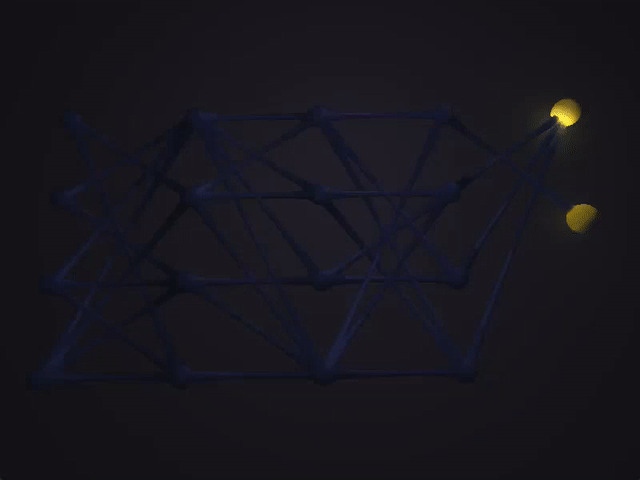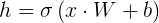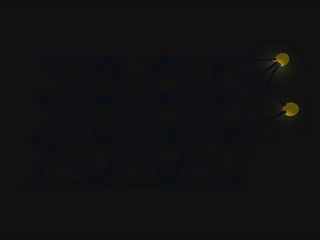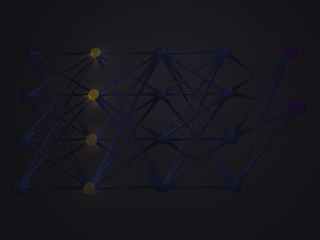# Introduction

This project is a 3D simulation of an artificial neural network. Artificial neural networks are modeled after the functionality of neurons in the brain. It simulates the propagation of information through a classic feed-forward, fully connected network. The combination of many basic signals shared between dozens or more neurons allows for very complex behavior to arise. While seemingly abstract, the underlying principles are quite simple. Each neuron recieves input and, based on that input, produces its own output. In this simulation these output signals are simulated as pulses of light traveling along "synapses" between each neuron. The brightness of the light pulses represent their relative magnitude. The thickness of each synapse indicates how each of these signals is weighted by the neurons. In other words it shows which connections between neurons are the most important.

# Controls

#### Movement

• WASD to move camera
• Space and X to move up and down
• F to speed up a little
• C to speed up a lot

#### Lighting

• < to decrease brightness
• > to increase brightness
• G to toggle global light

#### Network Layouts

• B for binary operation networks (AND, Exact OR, and XOR)
• V for a full four layer network with random weights and biases
• M for a sparse four layer network with random weights and biases

#### Network Inputs

• 1-4 for different inputs to the neural network

#### Animation Speed

• 0 to stop animation
• - to decrease animation speed
• + to increase animation speed
• \ to set animation speed back to default

#### Propagation Styles

• L for linear propagation
• K for cosine based propagation
• J for sine based propagation

#### Propagation Modifiers

• U adds an exponent of 3
• I adds an exponent of 2
• O removes exponent
• P adds an exponent of 0.75
• [ adds an exponent of 0.5

# Features

• Fully Functional Artificial Neural Network
• Dynamic Lighting
• Signal Propagation
• Camera Control
• Time Control

# Neural Network Implementation

At the heart of the simulation is a neural network implementation given by the following equation:This equation represents the input and output of one full layer in the network and can be computed entirely with matrices. Rather than pulling a bulky mathematics library just for a basic matrix and three operations, I chose to implement my own basic matrix data structure. The three operations needed are simple matrix addition, matrix dot product, and the ability to apply an arbitrary function to each element of the matrix. With this creating a neural network simply require linking repeated copies of the above equation together for each layer in the network.

This implementation does not include any code for training a neural network, such as back propagation or stochastic gradient descent. All weights and biases are either hard coded (in the case of the binary operations) or generated randomly. This greatly simplifies the complexity of the underlying neural network implementation and allows the focus to be placed on rendering the network simulation.

## Dynamic LightingLighting is updated every render cycle to give the clean lighting effects as the signals propagate through the network. To mitigate the computational resources required to do this many calculations are precomputed and stored in memory. For example the position of all the neurons as well as the position and rotation of all connections between the neurons are computed once on initialization and never again. This means the majority of the CPU resources are spent computing the dynamic light positions rather than recalculating the positions of static elements. To reduce the strain on the GPU, objects far from the current signals are never even told about the lights to begin with. For example neurons in the last layer of a network don't compute lighting if the lights are at the first layer. While doing all of this doesn't always guarantee perfect 60 FPS with 16+ moving light sources (at least on my 6 year old laptop) it typically maintains a satisfactory 30 FPS.

## Signal PropagationSignals in the network propagate through the network, carrying the output value of the previous layer to the next. However, how they traverse the connections between the neurons is highly subjective. Provided are three propagation styles. In the order of the images shown, there is linear propagation where the signal travels at a constant rate through the network. Next is a style based on a portion of a cosine curve which gives a flowing look and feel. Lastly there is a style based on a portion of a sine curve which gives a feeling the signals are being shot from one layer to the next. These propagation styles can be further manipulated with a set of propagation modifiers (see Controls).

## Time ControlsThe position of the signals as they propagate through the network is done so deterministically. A single value controls the exact position and brightness of every signal in the network. This allows for the signals to be manipulated in any way, such as forwards and backwards through the network as shown.

# Lesson Learned

This project is truly a lesson on just how expensive lighting is to compute. Granted this project does compute lighting the simple but expensive way it still shows just how impressive it is to see modern graphics produces stunningly complex scenes in realtime. It's hard to imagine that the orginal intent of this project was for a basic handwritten digit recognition neural network that would require hundreds and hundreds of lights.

# Resources

• Blender - Used to create the basic synapse model